# The Cubic Equation

Index

#### Introduction

In this page we discuss the problem of solving a cubic equation with real coefficients. In this case, the cubic equation has at least one real solution, and either none or two complex solutions. For readers familiar with the cubic formula, they will find different ways in which it can be written, and solving the cubic equation (that is, finding all the solutions of the equation) involves operations with complex numbers, even in the case that all solutions are real numbers. This fact certainly does not contribute to the popularization of the cubic formula. In this page we describe how to solve a cubic equation by only performing operations on real numbers, and when we find the complex number solutions we describe how to find the real and imaginary parts. In addition, we also describe how to use primitive cube roots of the unity to write solutions of the cubic equation using real radicals.

The classical way to solve a cubic equation is with one formula, or in practice three. The three formulas provide a way to find each solution, one at the time. We will also do that here. We will write three formulas to find the three solutions of a cubic equation, but will recognize that in order to do so we have to distinguish cases. The formula we use to find the three real solutions of a cubic equation is different from the formula we use to find the solutions of a cubic equation when there are two complex solutions. This analysis is summarized by separating the application of the formulas given here into cases as we discuss below.

Throughout this discussion we will talk about both the cubic equation ax3 + bx2+ cx + d = 0 and the corresponding cubic function f(x) = ax3 + bx2+ cx + d. Properties of the cubic function will illuminate the understanding of the solutions of the cubic equation.

#### Shape of the Graph of a Cubic Function

Before we go into details of each formula, we actually need to talk about cubic functions and understand how graphs are constructed in this case.

If one starts with a cubic function f(x) = ax3 + bx2 + cx + d, then when graphing such function we compute its derivative f'(x) = 3ax2 + 2bx + c in order to find the critical numbers. This can be done by solving the equation f'(x) = 0. The number of solutions of this equation depends on the discriminant of this quadratic given by D = (2b)2 - 4(3a)c = 4(b2 - 3ac). This leads us to the following table

 Case Explanation b2 - 3ac > 0 There are two critical numbers. The graph of f has a local maximum and a local minimum. b2 - 3ac = 0 There is only one critical number. The function is monotonic. There is one real root and two complex conjugate roots. The graph is obtained by translations and dilations of the graph of g(x) = x3 b2 - 3ac < 0 There are no critical numbers. The function is monotonic. The function has one real root and two complex conjugate roots.

Observe that in the case b2 - 3ac > 0 above we do not make a statement about the number of real and complex solutions. That is because the number of real and complex solutions is determined by another number.

#### The Discriminant

The discriminant of a cubic equation ax3 + bx2+ cx + d = 0 is defined as Δ = a4(α - β)2(α - γ)2(β - γ)2, where α, β, and γ, are solutions of the cubic equation.

It can be shown that the discriminant of the equation ax3 + bx2+ cx + d = 0 can be computed by the formula

Δ = b2c2 - 4ac3 - 4b3d + 18abcd - 27a2d2.

The interpretation of the discriminant is

 Case Explanation Δ > 0 The cubic equation has three different real solutions. Δ = 0 The cubic equation has a repeated solution. Δ < 0 There is only one real solution and two complex conjugate roots.

The discriminant of a cubic equation is related to the discriminant of the derivative of the associated cubic function by the inequality \begin{equation} 27a^{2}\Delta \leq 4(b^{2} - 3ac)^{3} \tag{1} \label{eq:inequality} \end{equation} This leads us to the following conclusions:

1. If b2 - 3ac < 0, then Δ < 0. This is consistent with the fact that if b2 - 3ac < 0, then the corresponding cubic function is monotonic, so it is one-to-one, implying that there is only one real solution of the cubic equation, which is what the statement Δ < 0 predicts.
2. If Δ > 0, then b2 - 3ac > 0. This says that if a cubic equation manages to have 3 solutions it does it by having a local maximum and a local minimum. This is not really enough to conclude that there are three solutions. In order to explain why there are three solutions in this case we need to know that if x1 and x2 are the critical numbers of the associated cubic function f, then \begin{equation}\label{critical}\tag{2} 27a^{2}f(x_{1})f(x_{2}) = -\Delta. \end{equation} It follows that since Δ > 0 the critical values of f must have opposite signs, which creates a solution between the critical points. The other two solutions can be predicted by comparing the location and sign of the critical values of the corresponding cubic function to the end behavior of such function.
The following image gives a graphical representation of these conclusions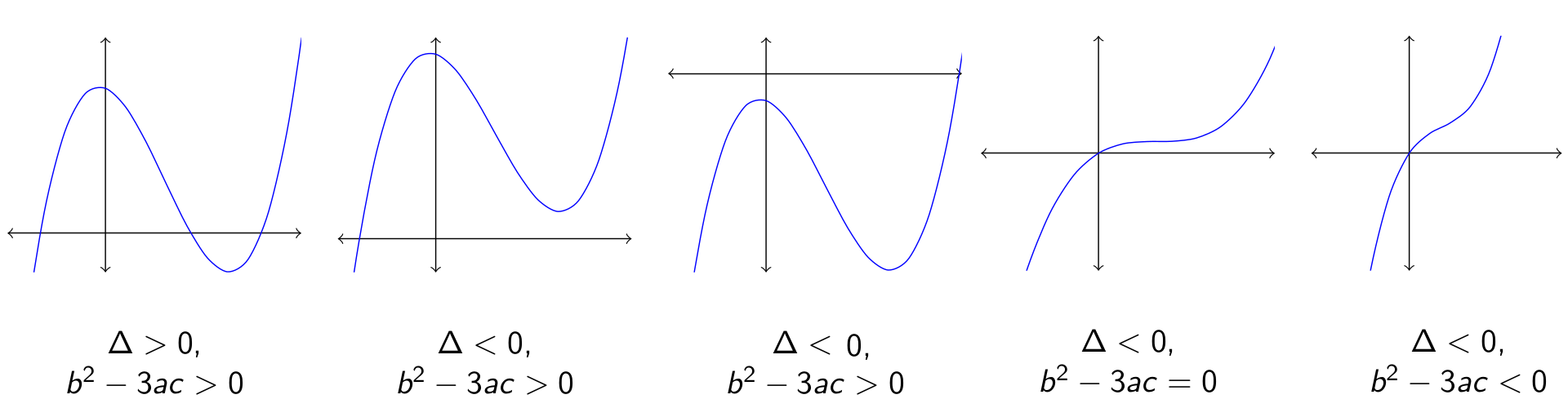The image above shows that the sign of the discriminant of the equation does not determine the sign of b2 - 3ac. The discriminant depends on the value of the coefficient d. Changing the value of d corresponds to a vertical shift in the graph of the corresponding cubic function. Since the discriminant of the derivative does not depend on d, the existence of a local minimum or a local maximum is not affected by these translations, but a vertical translation could put the two critical points in the graph either above or below the x-axis, making the product of the critical values of the corresponding function positive. This could make the equation change from having a positive discriminant with three real solutions to one having a negative discriminant with just one real solution, due to equation \eqref{critical} above.

This discussion can be summarized as follows. We divide the solution of the cubic equation into the following cases:

1. Case 1: b2 - 3ac = 0, when the graph of f is a transformation of the graph of x3.
2. Case 2: Δ = 0, when there are repeated roots.
3. Case 3: Δ > 0, when there are three different real roots.
4. Case 4: Δ < 0 and b2 - 3ac > 0, when there is only one real root and two critical points on the same side of the x-axis.
5. Case 5: b2 - 3ac < 0, when there is only one real root and no critical points. The function is monotonic.

#### The Inflection Point

Before we present the cubic formula in all different cases above we introduce one more ingredient in the solution. This ingredient appears naturally when we graph the corresponding cubic function. An inflection point is found by solving the equation f''(x) = 0. Since f''(x) = 3ax + b, the solution of f''(x) = 0 is $$x = -\frac{b}{3a},$$ and has ordinate $$y = f\left(-\frac{b}{3a}\right) = \frac{27a^{2}d + 2b^{3} - 9abc}{27a^{2}}.$$

The number that will appear in the solution of a cubic equation is 27a2y = 27a2d + 2b3 - 9abc.

The solution of the equation f''(x) = 0 we found above is an inflection point because f''(x) has a change in sign at that point and f is continuous there.

The formulas we present below contain both the abcissa and the ordinate of the inflection point of the cubic function. A better geometric explanation of what the terms in the formulas for solving the cubic equation mean will be given below.

### Formulas for Solving a Cubic Equation

We identified five cases in which we will solve the cubic equation. After they are presented we will discuss more about the meaning of the terms in each formula to get a sense of what the formulas say.

In this case the real solution of the equation ax3 + bx2 + cx + d = 0 is $$r_{k} = -\frac{b}{3a} + \omega^{k}\sqrt{\frac{b^{3}}{27a^{3}} - \frac{d}{a}},$$ where ω is primitive cube root of 1 and k = 0, or k = 1, or k = 2.

Case 2: Δ = 0. Repeated solutions.

We can assme that b2 - 3ac ≠ 0, because in the case that b2 - 3ac = 0 we can use the formula in case 1. Since b2 - 3ac ≠ 0, we must have b2 - 3ac > 0.

In this case, the double solution of the equation ax3 + bx2 + cx + d = 0 is given by $$r = \frac{9ad - bc}{2(b^{2} - 3ac)},$$ and the remaining solution is given by $$r = \frac{b}{a} - \frac{9ad - bc}{b^{2} - 3ac}.$$

Case 3: Δ > 0. Three real solutions.

Recall that in this case we must have b2 - 3ac > 0

The solutions of the equation ax3 + bx2 + cx + d = 0 are given by $$r_{k} = -\frac{b}{3a} + \frac{2\sqrt{b^{2} - 3ac}}{3a} \sin{\left(\frac{k\pi + (-1)^{k}\arcsin{\left(\frac{27a^{2}d + 2b^{3} - 9abc}{2(b^{2} - 3ac)^{3/2}}\right)}}{3}\right)},$$ where k = -1, or k = 0, or k = 1. In addition ar-1 < ar0 < ar1.

The real solution of the equation ax3 + bx2 + cx + d = 0 is given by $$x = -\frac{b}{3a} - \frac{2\lambda\sqrt{b^{2} - 3ac}}{3a} \cosh \left( \frac{\cosh^{-1}{(|p|)}}{3} \right),$$ where $$p = \frac{27a^{2}d + 2b^{3} - 9abc}{2(b^{2} - 3ac)^{3/2}},$$ and $$\lambda = \text{sign}{(27a^{2}d + 2b^{3} - 9abc)} = \text{sign}{(p)}.$$ The complex solutions are given by $$x = -\frac{b}{3a} + \frac{\lambda\sqrt{b^{2} - 3ac}}{3a} \left( \cosh \left( \frac{\cosh^{-1}{(|p|)}}{3} \right) \pm i\sqrt{3}\sinh \left( \frac{\cosh^{-1}{(|p|)}}{3} \right) \right).$$

The real solution of the equation ax3 + bx2 + cx + d = 0 is given by $$x = -\frac{b}{3a} - \frac{2\sqrt{|b^{2} - 3ac}|}{3a} \sinh \left( \frac{\sinh^{-1}{(p)}}{3} \right),$$ where $$p = \frac{27a^{2}d + 2b^{3} - 9abc}{2|b^{2} - 3ac|^{3/2}}.$$ The complex solutions are given by $$x = -\frac{b}{3a} + \frac{\sqrt{|b^{2} - 3ac|}}{3a} \left( \sinh \left( \frac{\sinh^{-1}{(p)}}{3} \right) \pm i\sqrt{3}\cosh \left( \frac{\sinh^{-1}{(p)}}{3} \right) \right).$$

### Discussion on the Form of the Cubic Formulas

Since the formulas for cases 1 and 2 above are for boundary cases we will refer the reader to the proof of these formulas for more details on their meanings. We are going to concentrate our discussion on cases 3 through 5.

#### Discussion about the Complex Solutions

Let us start our discussion by talking about cases 4 and 5. These are the cases in which there is only one real solution and two complex solutions. In both cases the formulas for finding the solutions have the form \begin{equation}\label{complex}\tag{3} \begin{array}{rcl} x_{1} & = & -\frac{b}{3a} - 2\rho \\ x_{2} & = & -\frac{b}{3a} + \rho + i\xi \\ x_{3} & = & -\frac{b}{3a} + \rho - i\xi\\ \end{array} \end{equation} for some real numbers ρ and ξ.

In these formulas, ρ and ξ depend on certain hyperbolic functions and their inverses. For example, if b2 - 3ac > 0 the number ρ depends on the hyperbolic cosine function and one-third of the inverse hyperbolic cosine of a number that depends on the ordinate of the inflection point and the cube of the square root of b2 - 3ac.

A similar comment can be made when b2 - 3ac < 0. In this case ρ depends on the hyperbolic sine function and one-third of the inverse hyperbolic sine of a number that depends on the ordinate of the inflection point and the cube of the square root of 3ac - b2.

In both cases the formula depends on the square root of |b2 - 3ac| and the ordinate of the inflection point of the graph of the corresponding cubic function. Specifically, these formulas depend on the number \begin{equation}\label{p}\tag{4} p = \frac{27a^{2}d + 2b^{3} - 9abc}{2|b^{2} - 3ac|^{3/2}} \end{equation}

In addition, in the case b2 - 3ac > 0 the number ρ depends on the sign of 27a2d + 2b3 - 9abc. This is the sign of the ordinate of the inflection point, what this sign reflects is that the real solution of the cubic equation is opposite of the sign of the ordinate of the inflection point, so if the inflection point is above the x-axis, the real solution is to the left of it, but if the inflection point is below the x-axis, the real solution is to the right of it. Compare this to the image below.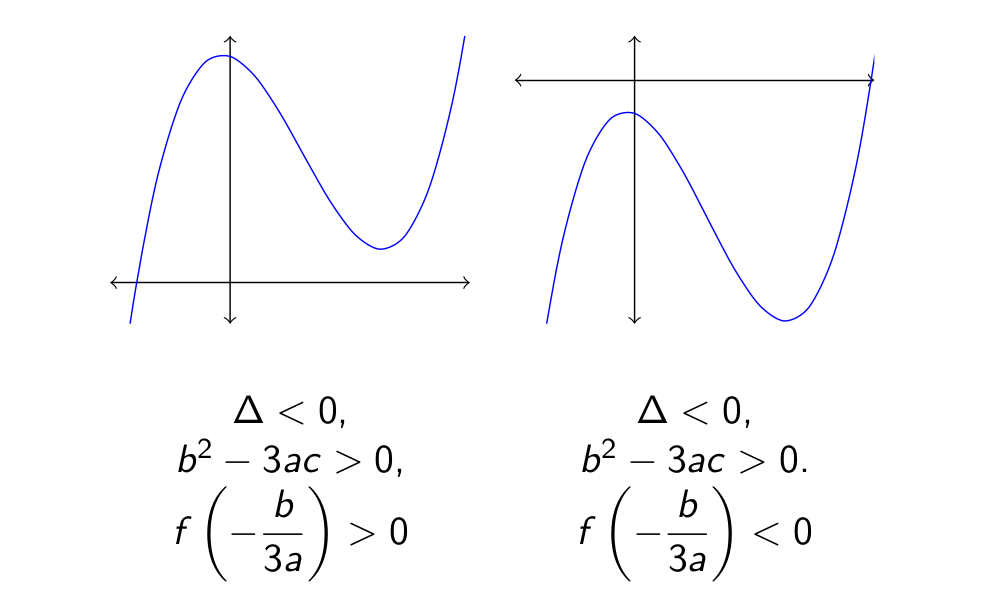The number ξ depends on the co-hyperbolic function of the hyperbolic function that appears in ρ. If the hyperbolic function in ρ is hyperbolic cosine, then the function in ξ is hyperbolic sine, and viceversa. The argument of the hyperbolic function in ξ is the same as argument in the hyperbolic function in ρ.

#### Geometry of the Complex Solutions

Since the formulas for the complex number solutions of the cubic equation depend on the hyperbolic cosine and hyperbolic sine of the same number, and these are coordinates of a point in a hyperbola, it is not surprising that the complex solutions of a cubic equation are in the hyperbola with equation $$\left(x + \frac{b}{3a}\right)^{2} - \frac{y^{2}}{3} = \frac{b^{2} - 3ac}{9a^{2}}.$$

This hyperbola has center at (-b/3a, 0) and asymptotes that make angles of 60 and 120 degrees with the positive direction of the x-axis.

If b2 - 3ac > 0 the hyperbola intersects the x-axis at the critical numbers of the corresponding cubic function. In the case that b2 - 3ac < 0 the hyperbola does not intersect the x-axis. In the graphs below the cubic function is plotted in blue, the corresponding hyperbola in red and the asymptotes in green.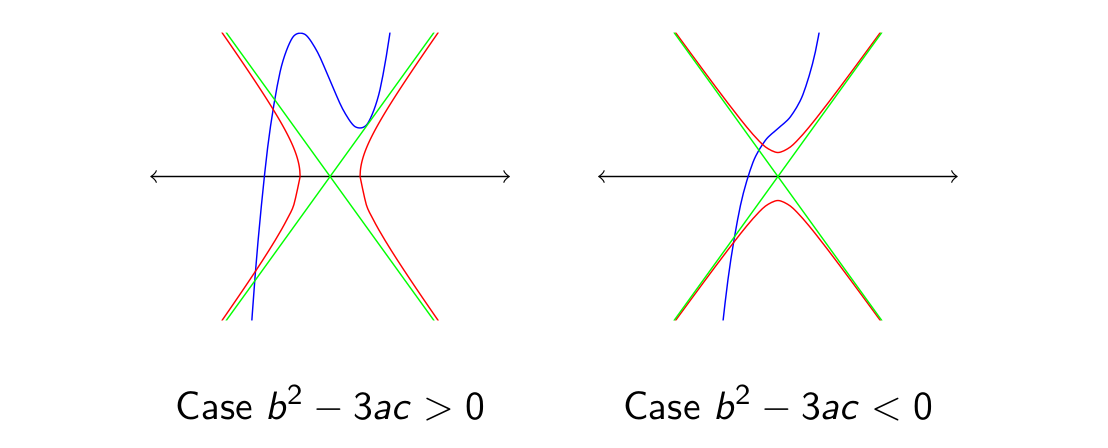The general form of the complex solutions in \eqref{complex} implies that the center of gravity of the triangle formed by the solutions is at the center of this hyperbola. Following this reasoning, the coefficient 2 next to the number ρ in the real solution and the coefficient 1 of the coefficient ρ in the complex solutions represents the fact that the center of gravity of a triangle divides a median in the 2:1 ratio. This means that the geometrical meaning of the number -b/3a in the cubic formula is that it represents the center of the hyperbola that contains two of the complex solutions of the equation.

In addition, the general form of the complex solutions in \eqref{complex} implies that the real and complex solutions are on opposite sides of the center of the hyperbola. If the real solution is to the left of the center of the hyperbola, the complex solutions are to the right of the center of the hyperbola, and viceversa. This provides a geometric way to find the complex number solutions of the equation given the real number solution.

#### The Geometry of the Three Real Solutions

In case 3 we showed how to solve a cubic equation that has three real solutions. The solution is expressed in terms on the sine and one-third of the inverse sine function of some angle, just like we found out in the case for solving the cubic equation when there are two complex number solutions. The three solutions are in three different regions, one is to the left of the leftmost critical points, another is between the critical points, and the latter one is to the right of the rightmost critical point. We can obtain each solution by setting the value of k to -1, 0, or 1. In the case that a > 0, following the increasing order of k also increases the solution we obtain.

One of then things we notice from the formulas in this case is that since |sin(α)| ≤ 1 for any angle α, then every solution rk must satisfy $$-\frac{b}{3a} - \frac{2\sqrt{b^{2} - 3ac}}{3a} \leq r_{k} \leq -\frac{b}{3a} + \frac{2\sqrt{b^{2} - 3ac}}{3a}$$ for any k in -1, 0, 1.

Later on we will refer to the left point of this interval as x3 and the right point of this interval as x4.

The formula for the solution in this case asks to trisect an angle whose sine is given by equation \eqref{p}. We can construct geometrically the angle that we need to trisect as follows. The inflection point of a cubic equation is halfway between the critical points of f. Recall that the critical points of f are at opposite sides of the x-axis, so a circle with center at the inflection point of the graph of f and radius the difference between the value of the local maximum and the ordinate of the inflection point will intersect the x-axis at two points, as the image below shows.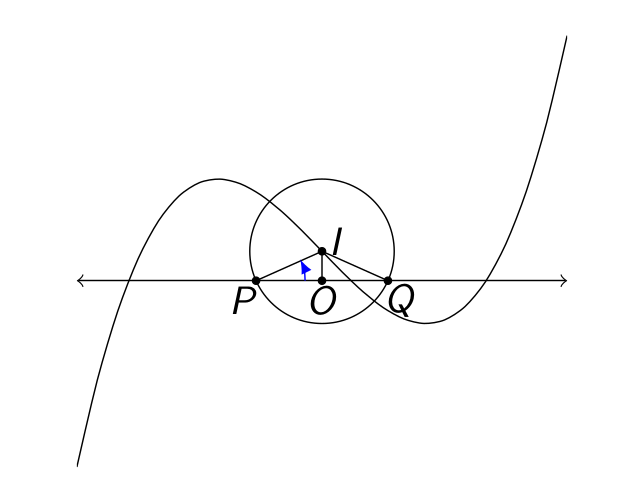In the image above I represents the inflection point of the graph. The points P and Q represent the points where the circle with center I and diameter the distance between the ordinate of the local maximum and the ordinate of the local minimum of f intersects the x-axis, and the point O corresponds to the orthogonal projection of I onto the x-axis

The acute angle that is being trisected in the formula for solving the cubic equation can be seen in the image above as the angle ∠ OPI measured counterclockwise. The sine of angle ∠ OPI is given by the number p in equality \eqref{p}.

Observe that in the case that there are three real solutions, and in the case that a > 0 the formula to solve the equation predicts that the solution x-1 is to the left of the inflection point, while it predicts that x1 is to the right of the inflection point. The solution x0 is to the right of the inflection point when this is above the x-axis and to the left of the inflection point when this is below the x-axis.

In the figure below we can see a process that leads geometrically from the solution between the critical points, called s0 below, to the other two solutions s-1 and s1, the lowest and bigest solution. Let us call I the abcissa of the inflection point of the graph and let us construct an ellipse with center at (-b/3a, 0) and equation $$\left(x + \frac{b}{3a}\right)^{2} + \frac{y^{2}}{3} = \frac{b^{2} - 3ac}{9a^{2}}.$$

Let us reflect the solution s0 across the abcissa I of the inflection point, at some point x so that I divides the segment from s0 to x in the 2:1 ratio. Then the vertical line through x intersects the ellipse at (x, y) and (x, -y). If we construct the circle with center (x, 0) and radius y then this circle intersects the x-axis at the solutions s-1 and s1.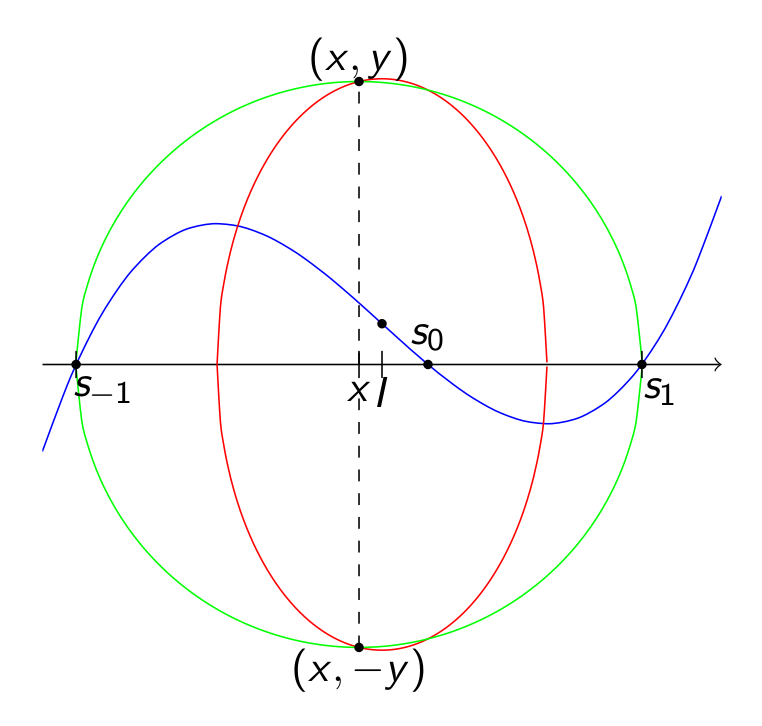From this geometric perspective the number -b/3a that appears in the formula to solve a cubic equation when there are three real solutions could represent the abcissa of the inflection point, because the circle with center at the inflection point is used to construct an angle which is needed to be trisected, or it could represent the center of the ellipse that leads to the other two solutions from the solution between the critical points. Whatever interpretation, the most important point is that there is geometry that explains the terms in the formulas for the solution of the cubic equation.

#### The Behavior of the Solutions when there are two Critical Points

Inequality \eqref{eq:inequality} does not predict the sign of Δ from knowing the sign of b2 - 3ac > 0. This can be explained by recalling that the discriminant depends on the coefficient d while the quatity b2 - 3ac does not. Since different values for d correspond to vertical translations of the graph of the cubic we can choose appropriate values of d so that Δ > 0, Δ = 0, or Δ < 0.

In order to understand what happens when we change the value of d let us assume that a > 0. In this case we know that f has a local maximum, say at x1, and a local minimum, say at x2, where x1 < x2. By increasing the value of d sufficiently we can move the local minimum value of f above the x-axis, which we have seen before makes Δ < 0 and therefore there will be a real root to the left of the inflection point, and two complex roots to the right of the inflection point on a hyperbola whose equation does not depend on d. Increasing further the value of d will make the real solution go towards negative infinity, while the two complex solutions will continue separating symmetrically further and further along the right branch of the hyperbola.

So let us assume now that we pick d sufficiently large so that the local minimum of f is above the x-axis, and let us see what happens with the three solutions of the corresponding cubic equation as we decrease d.

As we start decreasing d, we start with Δ < 0, and while we keep the local minimum above the x-axis, the real solution will start moving towards the right, while the complex solutions will start approaching each other. When the local minimum lands on the x-axis the equation will have a double root at x4 and therefore Δ will become 0. We can see this in the sequence of images below, where from left to right the graph is moved progressively down. The arrows indicate the direction in which the solutions are moving as we are moving the graph down. The real solutions appear as blue dots and the complex solutions appear as red dots.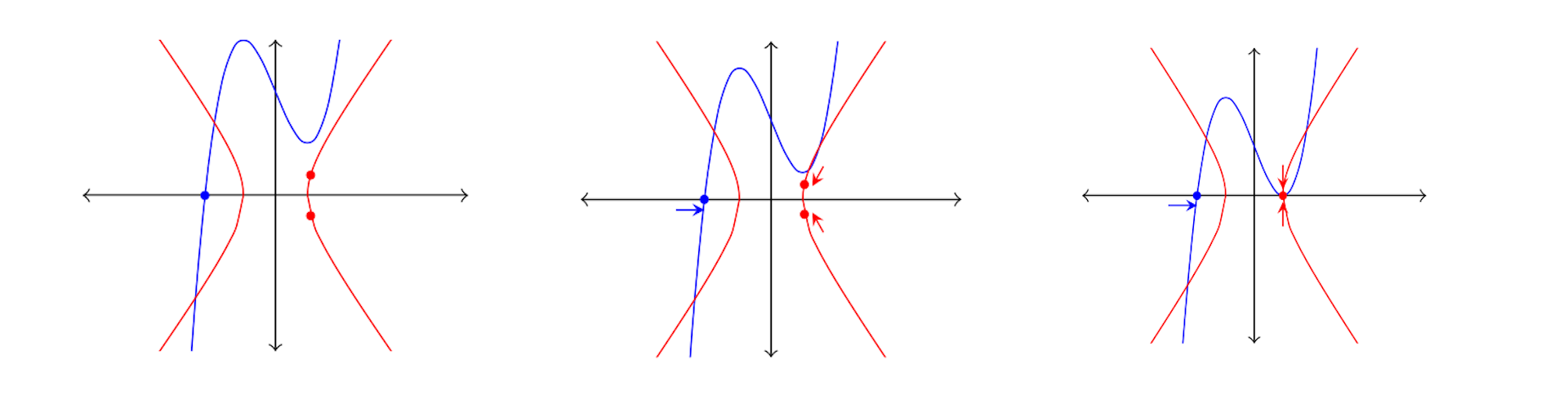At the moment that the graph of f touches the x-axis the real solution has been moving towards and now is landing on x3, that is the real solution lands at the leftmost value of any real solution when a cubic equation has three real solutions, and as we can see here, this is never reached when there are truly three different solutions.

One way to think about what happened so far is that the two complex solutions are travelling towards each other and at the time that the local minimum touches the x-axis they crash into each other becoming one real solution. As we keep decreasing d these solutions split apart, one travels towards the left, the other towards the right. Meanwhile, the solution that was originally at x3 also continued moving towards the right. We are now in the region where Δ > 0 and there are three different real solutions of the cubic equation. As we keep decreasing d, but stay in the region where Δ > 0, the solution stemming from x3 and one of the solutions stemming from the crashing two complex solutions are now in a collision course. When we decrease d enough so that the local maximum touches the x-axis, both real solutions travelling towards each other will crash at the local maximum, while the other real solution that was moving towards the right will land at x4, the rightmost value of any real solution when there are three different real solution. We can see this in the sequence of images below, where from left to right the graph has been moved progressively down, and the real solutions are represented as blue dots.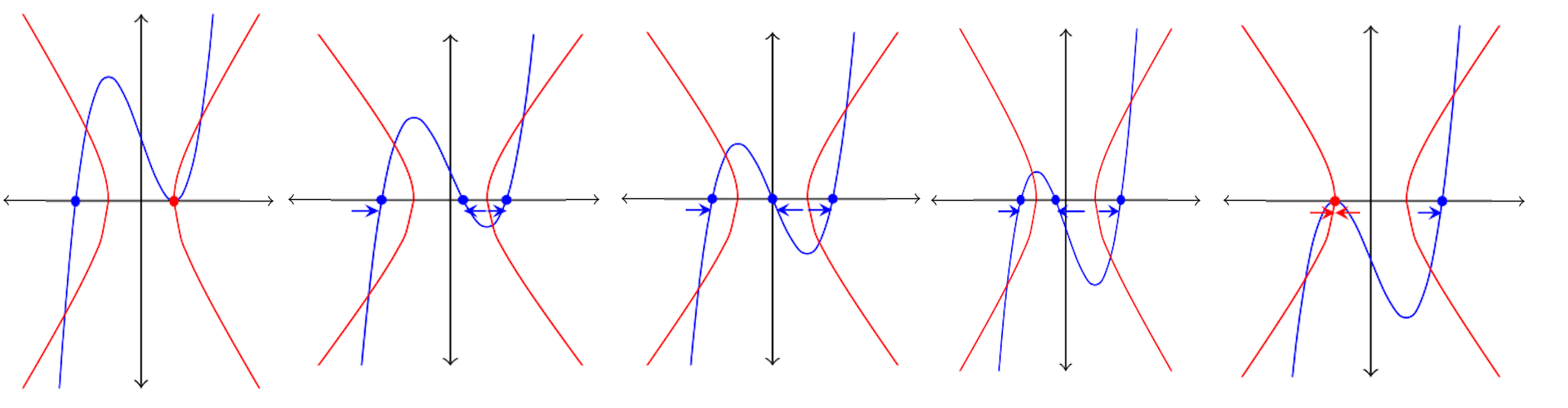Finally, as we keep decreasing d the local maximum becomes negative and we move to the region where Δ < 0. The two real solutions that had met at the critical point start to separate and become complex number solutions, one travelling up and another down symmetrically over the left branch of the hyperbola. The real solution that started at x4 will continue moving towards the right as we keep decreasing d. We can see this in the image below where the real solution is represented as a blue dot, and the complex solutions as red dots.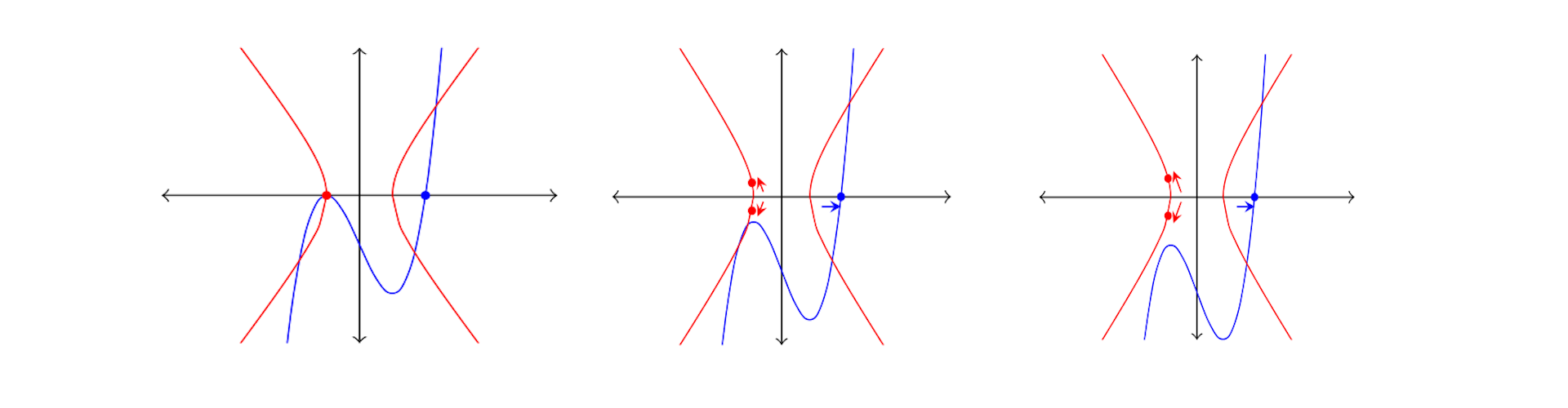The end points of the interval that holds the three real solutions, x3 and x4, correspond to the foci of the hyperbola that contains the complex solutions in the case that Δ < 0 and b2 - 3ac > 0.

The solutions are expressed in terms of primitive cube roots of the unity, namely $$\omega = \frac{-1 + i\sqrt{3}}{2}, \text{ or } \omega = \frac{-1 - i\sqrt{3}}{2}.$$
1. If b2 - 3ac < 0, then the three solutions are $$x_{k} = -\frac{b}{3a} - \frac{\omega^{k}\sqrt{\sqrt{-27a^{2}\Delta} + 27a^{2} + 2b^{3} - 9abc}} {3a\sqrt{2}} - \frac{\sqrt{2}(b^{2} - 3ac)\omega^{2k}} {3a\sqrt{\sqrt{-27a^{2}\Delta} + 27a^{2} + 2b^{3} - 9abc}},$$ where k ∈ {0, 1, 2}.
2. If Δ < 0 and b2 - 3ac > 0, then the three solutions are $$x_{k} = -\frac{b}{3a} + \frac{\lambda\omega^{k}\sqrt{\sqrt{-27a^{2}\Delta} + |27a^{2} + 2b^{3} - 9abc|}} {3a\sqrt{2}} + \frac{\lambda\sqrt{2}(b^{2} - 3ac)\omega^{2k}} {3a\sqrt{\sqrt{-27a^{2}\Delta} + |27a^{2} + 2b^{3} - 9abc|}},$$ where k ∈ {0, 1, 2} and λ = sign(27a2d + 2b3 - 9abc).